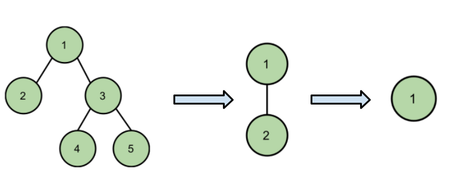# Jealous NobitaAhasan_1999CUET Intra University Jun...
Limits 10s, 1.0 GB · Custom Checker

Shizuka and Dekisugi are playing with a binary tree rooted at $1$. In each turn, the player chooses a subtree and erases it. The erased subtree must be a perfect binary tree. Shizuka takes the first turn. The player unable to move will lose the game.

Nobita wants to draw the initial binary tree for the game. Can Nobita draw the binary tree in such a way that Dekisugi will lose if both players play optimally?

Binary tree : A tree in which every node has at most two childs.

Perfect Binary tree : A binary tree in which all interior nodes have two children and all leaves have the same depth or same level. A single node is also a perfect binary tree.

Subtree : A subtree of a tree T is a tree consisting of a node in T and all of its descendants.

## Input

The first line contains an integer $T$ $\left(1 \leq T \leq 5\right)$ , the number of test cases.

Each test case contains an integer $N$ $\left(1 \leq N \leq 20\right)$, the number of nodes of the binary tree.

## Output

If Nobita cannot draw a binary tree, print “$\texttt{NO}$” (without quotes). Otherwise, print “$\texttt{YES}$” (without quotes) in a single line and in next $N-1$ lines print two integers $u,v$ $\left(1 \leq u, v \leq N\right)$ denoting the bidirectional edges of the binary tree. If there are multiple solutions then print any of them.

## Sample

InputOutput
2
5
2

YES
1 2
1 3
3 4
3 5
NO


$Case\ 1 :$ At first Shizuka removes the perfect binary tree rooted at $3$. Then Dekisugi removes node $2$. Finally Shizuka removes node $1$ . Dekisugi has no moves left so he loses.$Case\ 2 :$ There is only one possible construction of the binary tree having two nodes. Shizuka removes the perfect binary tree rooted at $2$. Then Dekisugi removes the only remaining node $1$. Shizuka has no move left so she loses.NumberTheory

### Submit